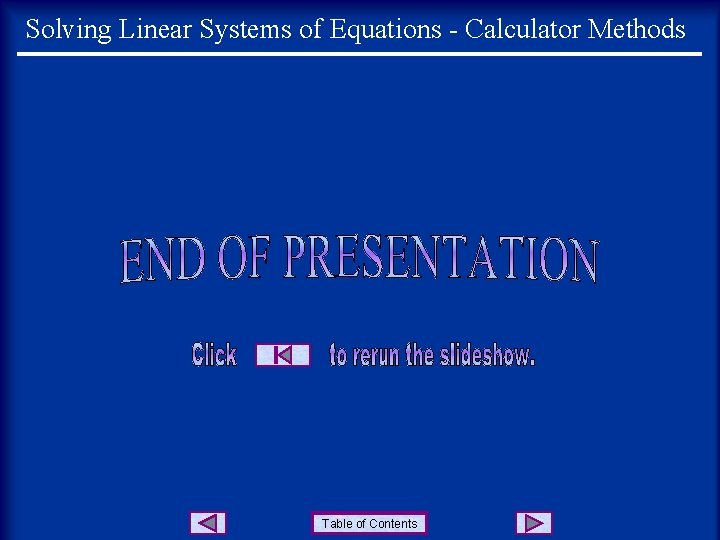# Solving Linear Systems of Equations Calculator Methods Consider

• Slides: 9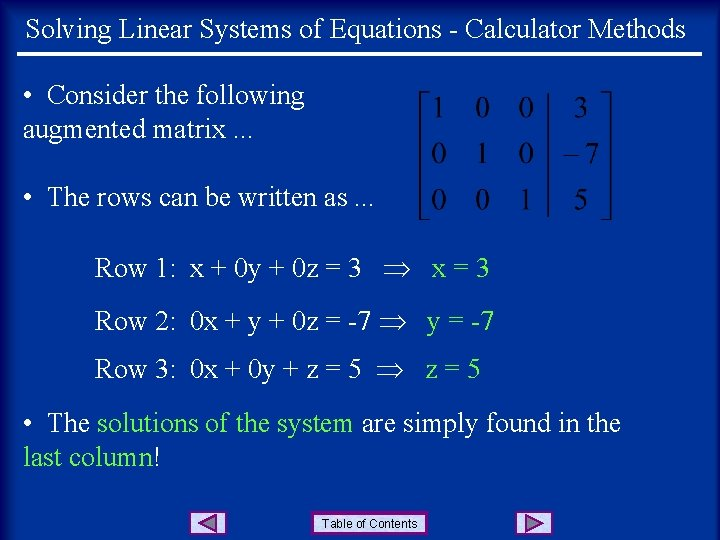Solving Linear Systems of Equations - Calculator Methods • Consider the following augmented matrix. . . • The rows can be written as. . . Row 1: x + 0 y + 0 z = 3 x = 3 Row 2: 0 x + y + 0 z = -7 y = -7 Row 3: 0 x + 0 y + z = 5 • The solutions of the system are simply found in the last column! Table of Contents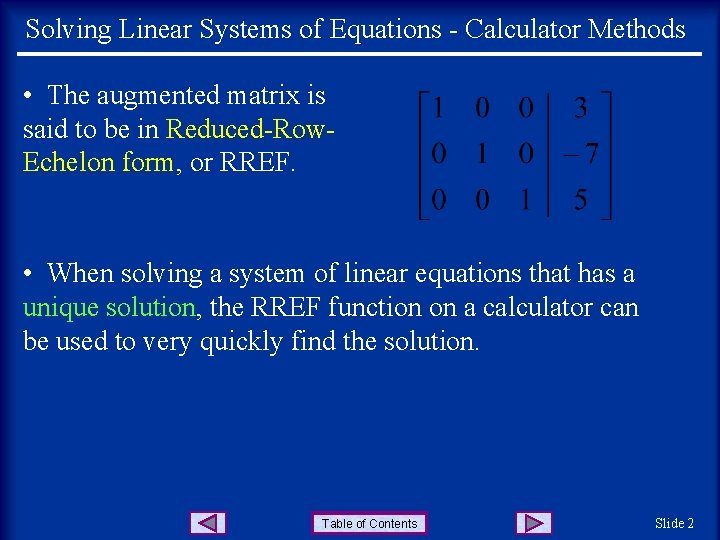Solving Linear Systems of Equations - Calculator Methods • The augmented matrix is said to be in Reduced-Row. Echelon form, or RREF. • When solving a system of linear equations that has a unique solution, the RREF function on a calculator can be used to very quickly find the solution. Table of Contents Slide 2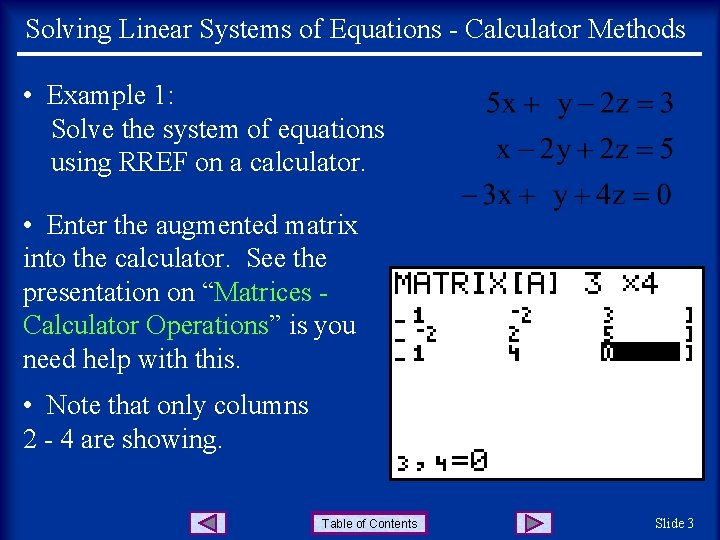Solving Linear Systems of Equations - Calculator Methods • Example 1: Solve the system of equations using RREF on a calculator. • Enter the augmented matrix into the calculator. See the presentation on “Matrices Calculator Operations” is you need help with this. • Note that only columns 2 - 4 are showing. Table of Contents Slide 3Solving Linear Systems of Equations - Calculator Methods • Press 2 nd|QUIT, then MATRIX and right arrow to MATH. . . • Go down in the list to rref( which is item B, and press ENTER. . . • This places rref( on the home screen. Now press MATRIX and ENTER to select matrix A. . . Table of Contents Slide 4Solving Linear Systems of Equations - Calculator Methods • Press ENTER. . . • The solutions to the system are found in the last column. To show the answers as fractions, press MATH|ENTER. . . Table of Contents Slide 4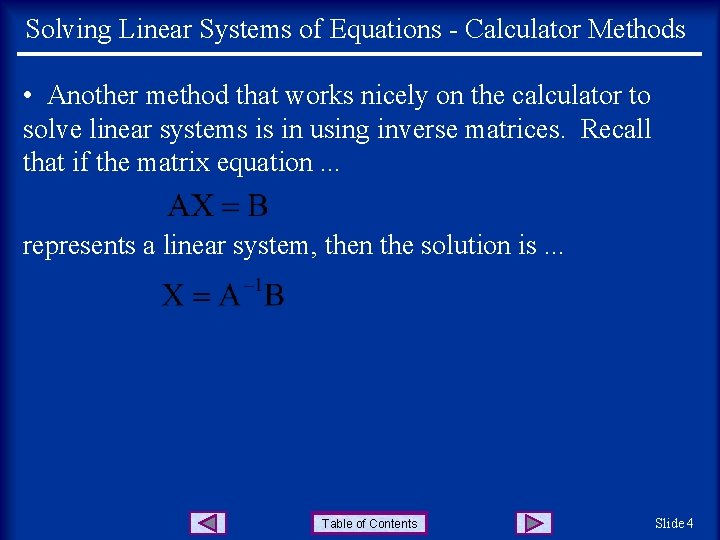Solving Linear Systems of Equations - Calculator Methods • Another method that works nicely on the calculator to solve linear systems is in using inverse matrices. Recall that if the matrix equation. . . represents a linear system, then the solution is. . . Table of Contents Slide 4Solving Linear Systems of Equations - Calculator Methods • Example 2: Solve the system of equations using an inverse matrix on a calculator. • Write matrix A, the coefficients, into the calculator. . . • Write matrix B, the constants, into the calculator. . . Table of Contents Slide 4Solving Linear Systems of Equations - Calculator Methods • Press 2 nd Quit and then MATRIX|ENTER to place matrix A on the home screen. . . • Press the x-1 key, then MATRIX|2 to place matrix B on the home screen. . . • Press ENTER. . . and the solutions are listed. • To write the solutions as fractions, press MATH|ENTER. . . Table of Contents Slide 4